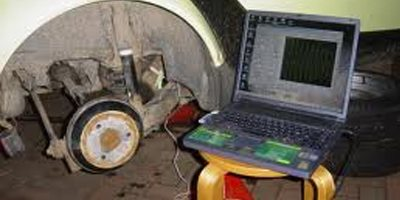# FREQUENCY MEASUREMENTS USING PC

This circuit uses parallel port of a computer for data input from binary counters. This data is used for measuring time and calculating the frequency of the signal. - Probir Goyal

4268PROBIR GOYAL
Here is a simple technique for measuring frequencies over quite a wide frequency range and with acceptable accuracy limits using a PC. It follows the basic technique of measuring low frequencies, i.e. at low frequency, period is measured for a complete wave and frequency is calculated from the measured time-period.

Cascaded binary counters are used for converting the high-frequency signals into low-frequency signals. The parallel port of a computer is used for data input from binary counters. This data is used for measuring time and calculating the frequency of the signal.

The block diagram shows the basic connections of the counters and parallel port pin numbers on 25-pin ‘D’ connector of a PC (control register 379 Hex is used for input). External hardware is used only for converting the higher frequency signals into low frequency signals. Thus, the major role in frequency-measurement is played by the software.

The PC generates a time-interrupt at a frequency of 18.21 Hz, i.e. after every 54.92 millisecond. Software uses this time-interrupt as a time-reference. The control register of the PC’s parallel port is read and the data is stored continuously in an array for approximately 54.9 ms using a loop. This stored data is then analysed bit-wise. Initially, the higher-order bit (MSB or the seventh-bit) of every array element is scanned for the presence of a complete square wave. If it is found, its time period is measured and if not then the second-highest order bit (sixth bit) is scanned. This operation is performed till the third bit and if no full square wave is still found, an error message is generated which indicates that either there is an error in reading or the frequency signal is lower than 19 Hz.

Lower three bits of the control register are not used. When a wave is found, along with its time-period and frequency components, its measurement precision in percentage is also calculated and displayed. Number of data taken in 54.9 ms is also displayed.As stated above, the lower starting range is about 19 Hz. Data is read for approximately 54.9 ms. Thus, the lowest possible frequency that can be measured is 1/.0549 Hz. Lower frequency range depends only on the sampling time and is practically fixed at 19 Hz (18.2 Hz, to be precise). Upper frequency range depends on factors such as value of the MOD counter used and the operating frequency range of the counter IC. If MOD-N counter is used (where N is an integer), upper limit (UL) of frequency is given by UL=19xN5 Hz. Thus for MOD 16 counters UL≈20 MHz, and for MOD 10 counters UL≈1.9 MHz. Care should be taken to ensure that this upper limit is within the operating frequency range of counter IC used. Precision of measurement is a machine-dependent parameter. High-speed machines will have better precision compared to others. Basically, precision depends directly upon the number of data read in a standard time. Precision of measurement varies inversely as the value of MOD counter used. Precision is high when MOD 10 counters are used in place of MOD 16 counters, but this will restrict the upper limit of frequency measurement and vice-versa.